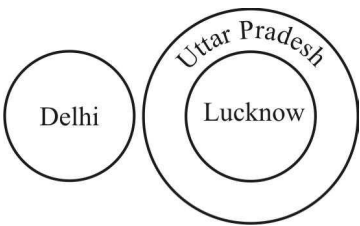## Logical Venn Diagram

#### Logical Venn Diagram

1. Which one of the following diagrams represents the correct relationship among 'Judge', 'Thief' and 'Criminal'?

1. On the basis of above given question , we get
Judge is different from both the thief and criminal. The thief comes under the class criminal.

##### Correct Option: C

On the basis of above given question , we get
Judge is different from both the thief and criminal. The thief comes under the class criminal.
From above it is clear that 'Judge' belongs to other class but 'Thief' and 'Criminal' belong to same classes .It represents a relationship in given figure .
Therefore , Option ( C ) is correct answer .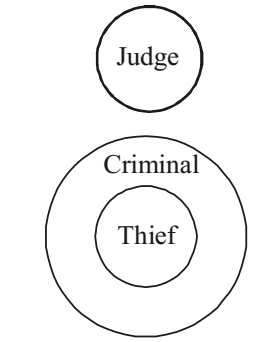1. Which of the answer figure indicates the best relationship between milk, goat, cow, hen?

1. According to question ,
we get the best relationship between milk, goat, cow and hen .As shown in given figure .

##### Correct Option: C

According to question ,
Goat is different from cow . But both give milk . So, we get the best relationship between milk, goat, cow and hen .As shown in given figure .
Therefore , required answer is option ( C ).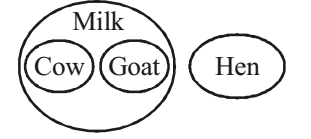1. Which figure represents the relationship among Sun, Moon, Molecule?

1. According to question , we can say that
Every thing is composed ofmolecules.
Sun is different from Moon.

##### Correct Option: D

According to question , we can say that
Every thing is composed ofmolecules.
Sun is different from Moon.
Therefore , required answer will be option ( D ).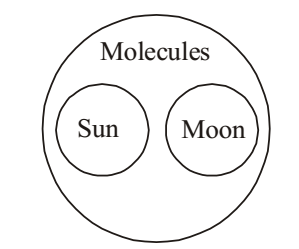1. Which one of the following diagrams best depicts the relationship among Earth, Sea, Sun ?

1. As per the given above question ,we can see that
Sea is a part of Earth . Sun is the star .

##### Correct Option: C

As per the given above question ,we can see that
Sea is a part of Earth . Sun is the star .
From above it is clear that Earth and Sea belong to same class but Sun belongs to different class .
Hence , required answer is option ( C ) .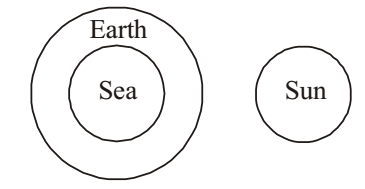1. Which one of the following diagrams represents the relationship among Delhi, Lucknow, Uttar Pradesh?

1. As we know that
Delhi is separate state while Lucknow is part of Uttar Pradesh.

##### Correct Option: C

As we know that
Delhi is separate state while Lucknow is part of Uttar Pradesh.
From above it is clear that Lucknow and Uttar Pradesh belong to same class and Delhi belongs to other class.
Hence , required answer will be option ( C ) .